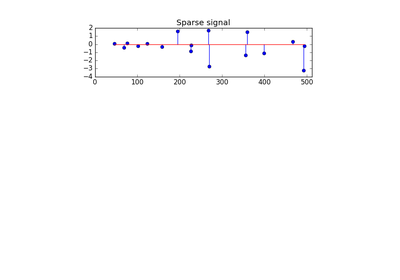# 3.2.4.1.8. `sklearn.linear_model`.OrthogonalMatchingPursuitCV¶

class `sklearn.linear_model.``OrthogonalMatchingPursuitCV`(copy=True, fit_intercept=True, normalize=True, max_iter=None, cv=None, n_jobs=1, verbose=False)[source]

Cross-validated Orthogonal Matching Pursuit model (OMP)

Parameters: copy : bool, optional Whether the design matrix X must be copied by the algorithm. A false value is only helpful if X is already Fortran-ordered, otherwise a copy is made anyway. fit_intercept : boolean, optional whether to calculate the intercept for this model. If set to false, no intercept will be used in calculations (e.g. data is expected to be already centered). normalize : boolean, optional, default False If True, the regressors X will be normalized before regression. This parameter is ignored when fit_intercept is set to False. When the regressors are normalized, note that this makes the hyperparameters learnt more robust and almost independent of the number of samples. The same property is not valid for standardized data. However, if you wish to standardize, please use preprocessing.StandardScaler before calling fit on an estimator with normalize=False. max_iter : integer, optional Maximum numbers of iterations to perform, therefore maximum features to include. 10% of `n_features` but at least 5 if available. cv : int, cross-validation generator or an iterable, optional Determines the cross-validation splitting strategy. Possible inputs for cv are: None, to use the default 3-fold cross-validation, integer, to specify the number of folds. An object to be used as a cross-validation generator. An iterable yielding train/test splits. For integer/None inputs, `KFold` is used. Refer User Guide for the various cross-validation strategies that can be used here. n_jobs : integer, optional Number of CPUs to use during the cross validation. If `-1`, use all the CPUs verbose : boolean or integer, optional Sets the verbosity amount Read more in the :ref:`User Guide `. : intercept_ : float or array, shape (n_targets,) Independent term in decision function. coef_ : array, shape (n_features,) or (n_features, n_targets) Parameter vector (w in the problem formulation). n_nonzero_coefs_ : int Estimated number of non-zero coefficients giving the best mean squared error over the cross-validation folds. n_iter_ : int or array-like Number of active features across every target for the model refit with the best hyperparameters got by cross-validating across all folds.

Methods

 `decision_function`(\*args, \*\*kwargs) DEPRECATED: and will be removed in 0.19. `fit`(X, y) Fit the model using X, y as training data. `get_params`([deep]) Get parameters for this estimator. `predict`(X) Predict using the linear model `score`(X, y[, sample_weight]) Returns the coefficient of determination R^2 of the prediction. `set_params`(\*\*params) Set the parameters of this estimator.
`__init__`(copy=True, fit_intercept=True, normalize=True, max_iter=None, cv=None, n_jobs=1, verbose=False)[source]
`decision_function`(*args, **kwargs)[source]

DEPRECATED: and will be removed in 0.19.

Decision function of the linear model.

Parameters: X : {array-like, sparse matrix}, shape = (n_samples, n_features) Samples. C : array, shape = (n_samples,) Returns predicted values.
`fit`(X, y)[source]

Fit the model using X, y as training data.

Parameters: X : array-like, shape [n_samples, n_features] Training data. y : array-like, shape [n_samples] Target values. self : object returns an instance of self.
`get_params`(deep=True)[source]

Get parameters for this estimator.

Parameters: deep : boolean, optional If True, will return the parameters for this estimator and contained subobjects that are estimators. params : mapping of string to any Parameter names mapped to their values.
`predict`(X)[source]

Predict using the linear model

Parameters: X : {array-like, sparse matrix}, shape = (n_samples, n_features) Samples. C : array, shape = (n_samples,) Returns predicted values.
`score`(X, y, sample_weight=None)[source]

Returns the coefficient of determination R^2 of the prediction.

The coefficient R^2 is defined as (1 - u/v), where u is the regression sum of squares ((y_true - y_pred) ** 2).sum() and v is the residual sum of squares ((y_true - y_true.mean()) ** 2).sum(). Best possible score is 1.0 and it can be negative (because the model can be arbitrarily worse). A constant model that always predicts the expected value of y, disregarding the input features, would get a R^2 score of 0.0.

Parameters: X : array-like, shape = (n_samples, n_features) Test samples. y : array-like, shape = (n_samples) or (n_samples, n_outputs) True values for X. sample_weight : array-like, shape = [n_samples], optional Sample weights. score : float R^2 of self.predict(X) wrt. y.
`set_params`(**params)[source]

Set the parameters of this estimator.

The method works on simple estimators as well as on nested objects (such as pipelines). The latter have parameters of the form `<component>__<parameter>` so that it’s possible to update each component of a nested object.

Returns: self :

## 3.2.4.1.8.1. Examples using `sklearn.linear_model.OrthogonalMatchingPursuitCV`¶Orthogonal Matching Pursuit